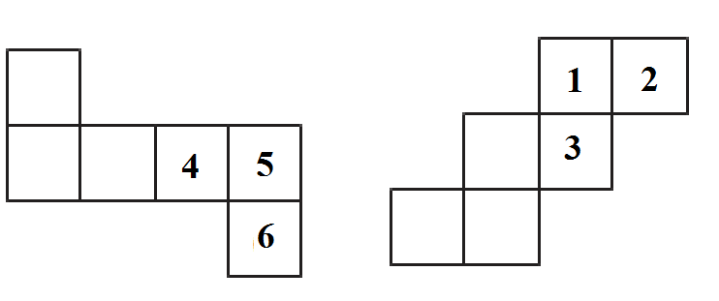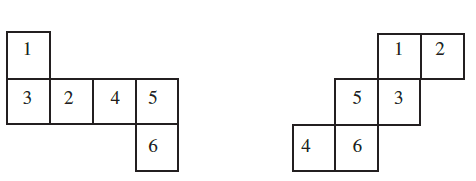# Ex.15.1 Q2 Visualizing Solid Shapes - NCERT Maths Class 7

Go back to  'Ex.15.1'

## Question

Dice are cubes with dots on each face. Opposite faces of a die always have a total of seven dots on them.

Here are two nets to make dice (cubes); the numbers inserted in each square indicate the number of dots in that box.Insert suitable numbers in the blanks, remembering that the number on the opposite faces should total to $$7$$.

## Text Solution

What is known?

Two nets of a dice on which number of dots on some faces are given.

What is unknown?

Number in the squares where there are blanks.

Reasoning:

As we know dice are cubes with dots on each face. Opposite faces of a die always have a total of seven dots on them. Also, in this question nets to make dice (cubes) is given along the numbers inserted in each square indicate the number of dots in that box.

Steps:

In figure (i) $$1^{\rm st}$$ blank net will be number $$1$$ in dice because opposite net to it will be $$6$$ as given. $$2^{\rm{nd}}$$ net will be $$3$$ as opposite to it will be $$4$$ as given and last $$3^\rm{rd}$$ net will be $$2$$ as opposite to it will be $$5$$.

In figure (ii) $$1^ \rm {st}$$ blank net will be no. $$5$$ in dice because opposite net to it will be $$2$$ as given. $$2^\rm{nd}$$ net will be $$6$$ as opposite to it will be $$4$$ as given and last $$3^\rm{rd}$$ net will be $$4$$ as opposite to it will be $$3$$.Learn from the best math teachers and top your exams

• Live one on one classroom and doubt clearing
• Practice worksheets in and after class for conceptual clarity
• Personalized curriculum to keep up with school